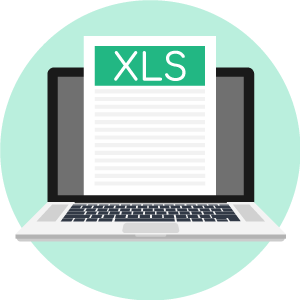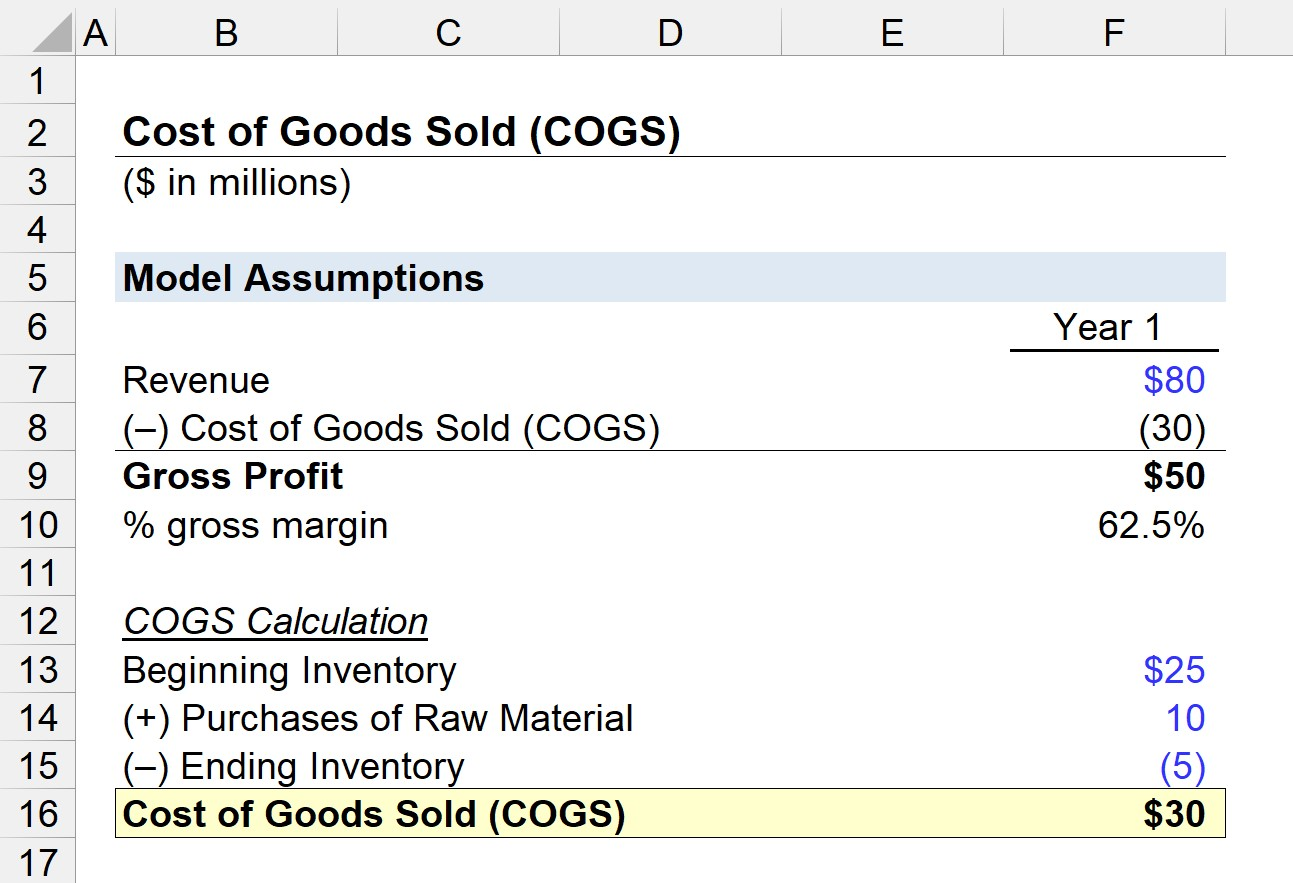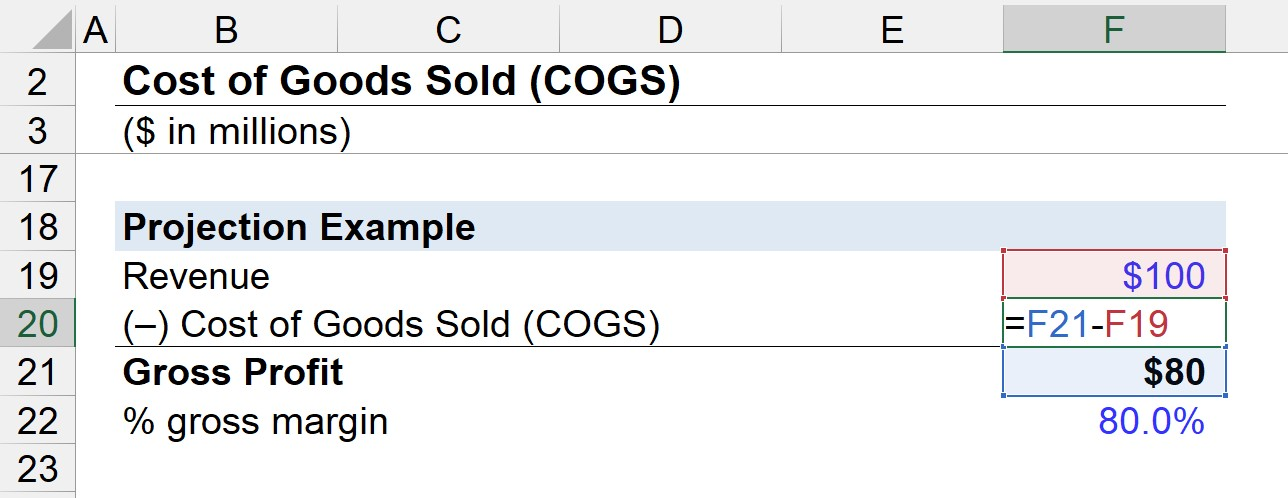# Cost of Goods Sold (COGS)

Understand the Definition of Cost of Goods Sold (COGS)• What does the cost of goods sold (COGS) metric represent?
• What is the formula for calculating COGS?
• How can COGS be evaluated to derive insights regarding pricing?
• Why is COGS an important metric to track?

## Cost of Goods Sold (COGS) Overview

The cost of goods sold (COGS) is the accounting term used to describe the direct expenses incurred to produce revenue.

On the income statement, the cost of goods sold (COGS) line item is the first expense following revenue (i.e. the “top line”).

 Examples of Cost of Goods Sold (COGS) Purchase of Inventory/Merchandise Cost of Raw Materials Cost of Direct Labor

The categorization of expenses into COGS or operating expenses (OpEx) is entirely dependent on the industry in question.

For instance, “Cost of Direct Labor” is recognized as COGS for service-oriented industries where the production of the company’s goods sold are directly related to labor.

But not all labor costs are recognized as COGS, which is why each company’s breakdown of their expenses and the process of revenue creation must be assessed.

As another industry-specific example, COGS for SaaS companies could include hosting fees and third-party APIs integrated directly into the selling process.

###### COGS vs Operating Expenses

Conversely, COGS excludes operating expenses – i.e. indirect costs – such as overhead costs, utilities, rent, and marketing expenses.

While a broad generalization, COGS tend to consist of variable costs, as the value is dependent on the production volume.

In contrast, OpEx tends to consist of fixed costs, which means the value remains relatively constant regardless of the level of production output.

For example, a company’s rental expense for a facility remains fixed based on a signed rental agreement.

## COGS and Gross Profit

The gross profit metric represents the earnings remaining once direct costs (i.e. COGS) is deducted from revenue.

### Gross Profit Formula

• Gross Profit = Revenue – Cost of Goods Sold (COGS)

The gross profit helps determine the portion of revenue that can be used for operating expenses (OpEx) as well as non-operating expenses like interest expense and taxes.

In addition, the gross profit of a company can be divided by revenue to arrive at the gross profit margin, which is among one of the most frequently used profit measures.

### Gross Margin Formula

• Gross Margin = (Revenue – COGS) / Revenue

For companies attempting to increase their gross margins, selling at higher quantities is one method to benefit from lower per-unit costs.

If a company orders more raw materials from suppliers, it can likely negotiate better pricing, which reduces the cost of raw materials per unit produced (and COGS).

## Interpreting COGS

Calculating the COGS of a company is important because it measures the real cost of producing a product, as only the direct cost have been subtracted.

In effect, the company gets a better sense of the cost of producing the good or providing the service – and thereby can price their offerings better.

Generally speaking, COGS will grow alongside revenue because theoretically, the more product/service sold, the more must be spent for production.

But of course, there are exceptions, since COGS varies depending on a company’s particular business model.

###### Cost/Revenue Matching Principle

Under the matching principle of accrual accounting, each cost must be recognized in the same period as when the revenue was earned.

For instance, just the costs associated with the inventory sold in the current period can be recognized on the income statement, which is where the LIFO vs FIFO inventory accounting methods can be a source of debate.

## Cost of Goods Sold (COGS) Formula

The calculation of COGS is distinct in that each expense is not just added together, but rather, the beginning balance is adjusted for the cost of inventory purchased and the ending inventory.

### COGS Formula

• COGS = Beginning Inventory + Purchases in the Current Period – Ending Inventory
• Beginning Inventory – The amount of inventory rolled over (i.e. leftover) from the prior period
• Purchases in Current Period – Cost of purchases made during the current period
• Ending Inventory – Inventory NOT sold during the current period

## COGS Excel Template

Now that we’ve defined the cost of goods sold (COGS) line item, we can complete a simple modeling exercise in Excel. Fill out the form below to access the file:Submitting ...

## Cost of Goods Sold (COGS) Example Calculation

Let’s say there’s a clothing retail store that starts off Year 1 with \$25 million in beginning inventory, which is the ending inventory balance from the prior year.

Throughout Year 1, the retailer purchases \$10 million in additional inventory and fails to sell \$5 million in inventory.

With that said, the COGS in Year 1 can be calculated with the following simple formula:

• COGS = \$25m + \$10m – \$5m = \$30m

The \$30 million in COGS is then linked back to the gross profit calculation, but with the sign flipped to show that it represents a cash outflow.

The gross profit is equal to \$50 million in Year 1 (\$80m – \$30m), while the gross margin is 62.5%.

• Gross Profit = \$80m – \$30m = \$50m
• Gross Margin = \$80m / \$50m = 62.5%## Example COGS Projection

To wrap up our post on COGS, we’ll conclude with a quick explanation of one forecasting approach of COGS often seen in financial models.

Since public companies are not obligated by the SEC to disclose confidential data regarding their internal inventory data, one method is to assume a gross margin based on historical (and industry) averages.

Here in our example, we assume a gross margin of 80.0%, which we’ll multiply by the revenue amount of \$100 million to get \$80 million as our gross profit.

In the final step, we subtract revenue from gross profit to arrive at – \$20 million as our COGS figure.Inline FeedbacksAmanda White
January 31, 2022 10:03 amLearn Financial Modeling Online

Everything you need to master financial and valuation modeling: 3-Statement Modeling, DCF, Comps, M&A and LBO.

X

The Wall Street Prep Quicklesson Series

7 Free Financial Modeling Lessons

Get instant access to video lessons taught by experienced investment bankers. Learn financial statement modeling, DCF, M&A, LBO, Comps and Excel shortcuts.